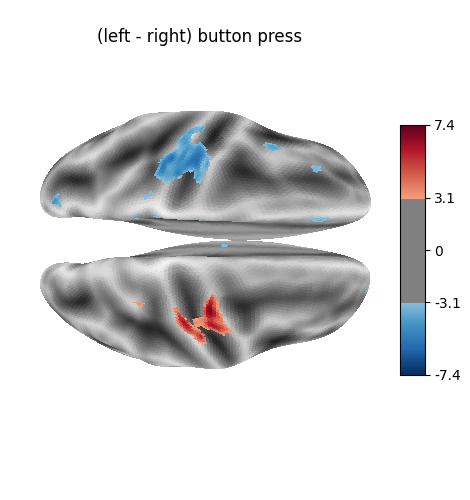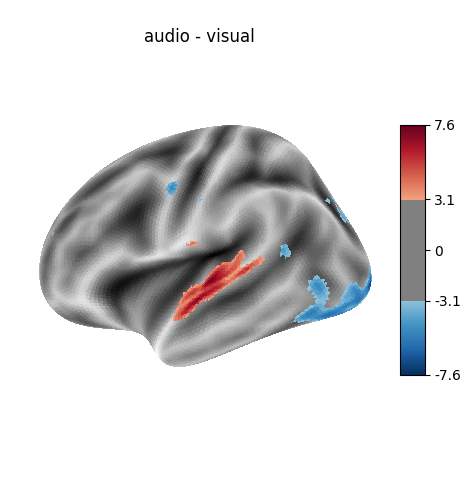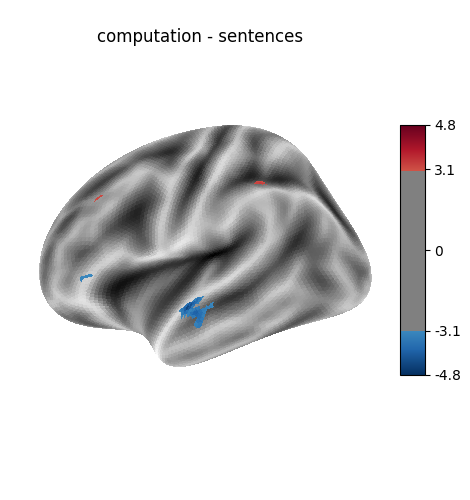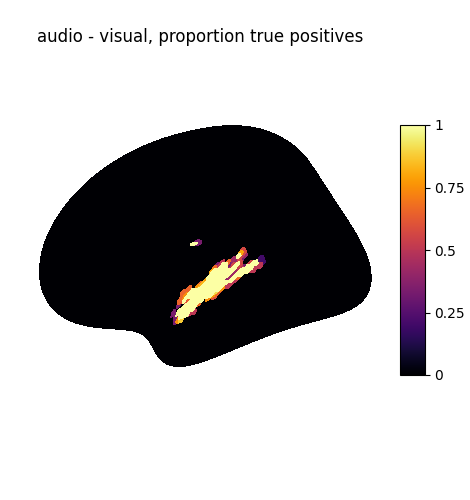# Example of surface-based first-level analysis#

A full step-by-step example of fitting a GLM to experimental data sampled on the cortical surface and visualizing the results.

More specifically:

1. A sequence of fMRI volumes is loaded.

2. fMRI data are projected onto a reference cortical surface (the FreeSurfer template, fsaverage).

3. A design matrix describing all the effects related to the data is computed.

4. A GLM is applied to the dataset (effect/covariance, then contrast estimation).

The result of the analysis are statistical maps that are defined on the brain mesh. We display them using Nilearn capabilities.

The projection of fMRI data onto a given brain mesh requires that both are initially defined in the same space.

• The functional data should be coregistered to the anatomy from which the mesh was obtained.

• Another possibility, used here, is to project the normalized fMRI data to an MNI-coregistered mesh, such as fsaverage.

The advantage of this second approach is that it makes it easy to run second-level analyses on the surface. On the other hand, it is obviously less accurate than using a subject-tailored mesh.

## Prepare data and analysis parameters#

Prepare the timing parameters.

```t_r = 2.4
slice_time_ref = 0.5
```

Prepare the data. First, the volume-based fMRI data.

```from nilearn.datasets import fetch_localizer_first_level

data = fetch_localizer_first_level()
fmri_img = data.epi_img
```

```import pandas as pd

events_file = data.events
```

## Project the fMRI image to the surface#

For this we need to get a mesh representing the geometry of the surface. We could use an individual mesh, but we first resort to a standard mesh, the so-called fsaverage5 template from the FreeSurfer software.

```import nilearn

fsaverage = nilearn.datasets.fetch_surf_fsaverage()
```

The projection function simply takes the fMRI data and the mesh. Note that those correspond spatially, are they are both in MNI space.

```from nilearn import surface

texture = surface.vol_to_surf(fmri_img, fsaverage.pial_right)
```

## Perform first level analysis#

This involves computing the design matrix and fitting the model. We start by specifying the timing of fMRI frames.

```import numpy as np

n_scans = texture.shape
frame_times = t_r * (np.arange(n_scans) + .5)
```

Create the design matrix.

We specify an hrf model containing the Glover model and its time derivative The drift model is implicitly a cosine basis with a period cutoff at 128s.

```from nilearn.glm.first_level import make_first_level_design_matrix

design_matrix = make_first_level_design_matrix(frame_times,
events=events,
hrf_model='glover + derivative'
)
```

Setup and fit GLM.

Note that the output consists in 2 variables: labels and fit. labels tags voxels according to noise autocorrelation. estimates contains the parameter estimates. We keep them for later contrast computation.

```from nilearn.glm.first_level import run_glm

labels, estimates = run_glm(texture.T, design_matrix.values)
```

## Estimate contrasts#

Specify the contrasts.

For practical purpose, we first generate an identity matrix whose size is the number of columns of the design matrix.

```contrast_matrix = np.eye(design_matrix.shape)
```

At first, we create basic contrasts.

```basic_contrasts = dict([(column, contrast_matrix[i])
for i, column in enumerate(design_matrix.columns)])
```

Next, we add some intermediate contrasts and one contrast adding all conditions with some auditory parts.

```basic_contrasts['audio'] = (
basic_contrasts['audio_left_hand_button_press']
+ basic_contrasts['audio_right_hand_button_press']
+ basic_contrasts['audio_computation']
+ basic_contrasts['sentence_listening'])

basic_contrasts['visual'] = (
basic_contrasts['visual_left_hand_button_press']
+ basic_contrasts['visual_right_hand_button_press']
+ basic_contrasts['visual_computation']

# one contrast adding all conditions involving computation
basic_contrasts['computation'] = (basic_contrasts['visual_computation']
+ basic_contrasts['audio_computation'])

# one contrast adding all conditions involving sentences
basic_contrasts['sentences'] = (basic_contrasts['sentence_listening']
```

Finally, we create a dictionary of more relevant contrasts

• ‘left - right button press’: probes motor activity in left versus right button presses.

• ‘audio - visual’: probes the difference of activity between listening to some content or reading the same type of content (instructions, stories).

• ‘computation - sentences’: looks at the activity when performing a mental computation task versus simply reading sentences.

Of course, we could define other contrasts, but we keep only 3 for simplicity.

```contrasts = {
'left - right button press': (
basic_contrasts['audio_left_hand_button_press']
- basic_contrasts['audio_right_hand_button_press']
+ basic_contrasts['visual_left_hand_button_press']
- basic_contrasts['visual_right_hand_button_press']
),
'audio - visual': basic_contrasts['audio'] - basic_contrasts['visual'],
'computation - sentences': (
basic_contrasts['computation']
- basic_contrasts['sentences']
)
}
```

Let’s estimate the contrasts by iterating over them.

```from nilearn import plotting
from nilearn.glm.contrasts import compute_contrast

for index, (contrast_id, contrast_val) in enumerate(contrasts.items()):
print('  Contrast % i out of %i: %s, right hemisphere' %
(index + 1, len(contrasts), contrast_id))
# compute contrast-related statistics
contrast = compute_contrast(labels, estimates, contrast_val,
contrast_type='t')
# we present the Z-transform of the t map
z_score = contrast.z_score()
# we plot it on the surface, on the inflated fsaverage mesh,
# together with a suitable background to give an impression
# of the cortex folding.
plotting.plot_surf_stat_map(
fsaverage.infl_right, z_score, hemi='right',
title=contrast_id, colorbar=True,
threshold=3., bg_map=fsaverage.sulc_right)
```
•••```Contrast  1 out of 3: left - right button press, right hemisphere
Contrast  2 out of 3: audio - visual, right hemisphere
Contrast  3 out of 3: computation - sentences, right hemisphere
```

## Analysing the left hemisphere#

Note that re-creating the above analysis for the left hemisphere requires little additional code!

We project the fMRI data to the mesh.

```texture = surface.vol_to_surf(fmri_img, fsaverage.pial_left)
```

Then we estimate the General Linear Model.

```labels, estimates = run_glm(texture.T, design_matrix.values)
```

Finally, we create contrast-specific maps and plot them.

```for index, (contrast_id, contrast_val) in enumerate(contrasts.items()):
print('  Contrast % i out of %i: %s, left hemisphere' %
(index + 1, len(contrasts), contrast_id))
# compute contrasts
contrast = compute_contrast(labels, estimates, contrast_val,
contrast_type='t')
z_score = contrast.z_score()
# plot the result
plotting.plot_surf_stat_map(
fsaverage.infl_left, z_score, hemi='left',
title=contrast_id, colorbar=True,
threshold=3., bg_map=fsaverage.sulc_left)

plotting.show()
```
•••```Contrast  1 out of 3: left - right button press, left hemisphere
Contrast  2 out of 3: audio - visual, left hemisphere
Contrast  3 out of 3: computation - sentences, left hemisphere
```

Total running time of the script: ( 0 minutes 19.934 seconds)

Estimated memory usage: 209 MB

Gallery generated by Sphinx-Gallery